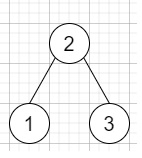# Binary Tree Longest Consecutive Sequence II in C++

Suppose we have a binary tree; we have to find the length of the Longest Consecutive Path in that Binary Tree. Here the path can be either increasing or decreasing. So as an example [1,2,3,4] and [4,3,2,1] are both considered as a valid path, but the path [1,2,4,3] is not a valid one.

Otherwise, the path can be in the child-Parent-child sequence, where not necessarily be parent-child order.

So, if the input is likethen the output will be 3 as the longest consecutive path will be like [1, 2, 3] or [3, 2, 1].

To solve this, we will follow these steps −

• Define a function solveUtil(), this will take node,

• if the node is null, then −

• return { 0, 0 }

• left = solveUtil(left of node)

• left = solveUtil(right of node)

• Define one pair temp := {1,1}

• if left of node is present and value of left of node is same as value of node + 1, then −

• temp.first := maximum of temp.first and 1 + left.first

• ans := maximum of ans and temp.first

• if right of node is present and value of right of node is same as value of node + 1, then −

• temp.first := maximum of temp.first and 1 + right.first

• ans := maximum of ans and temp.first

• if left of node is present and value of left of node is same as value of node - 1, then −

• temp.second := maximum of temp.second and 1 + left.second

• ans := maximum of ans and temp.second

• if right of node is present and value of right of node is same as value of node - 1, then −

• temp.second := maximum of temp.second and 1 + right.second

• ans := maximum of ans and temp.second

• ans := maximum of { ans and temp.first + temp.second - 1 }

• return temp

• From the main method do the following −

• ans := 0

• solveUtil(root)

• return ans

## Example

Let us see the following implementation to get a better understanding −

Live Demo

#include <bits/stdc++.h>
using namespace std;
class TreeNode{
public:
int val;
TreeNode *left, *right;
TreeNode(int data){
val = data;
left = NULL;
right = NULL;
}
};
class Solution {
public:
int ans = 0;
pair<int, int> solveUtil(TreeNode* node){
if (!node) {
return { 0, 0 };
}
pair<int, int> left = solveUtil(node->left);
pair<int, int> right = solveUtil(node->right);
pair<int, int> temp = { 1, 1 };
if (node->left && node->left->val == node->val + 1) {
temp.first = max(temp.first, 1 + left.first);
ans = max(ans, temp.first);
}
if (node->right && node->right->val == node->val + 1) {
temp.first = max(temp.first, 1 + right.first);
ans = max(ans, temp.first);
}
if (node->left && node->left->val == node->val - 1) {
temp.second = max(temp.second, 1 + left.second);
ans = max(ans, temp.second);
}
if (node->right && node->right->val == node->val - 1) {
temp.second = max(temp.second, 1 + right.second);
ans = max(ans, temp.second);
}
ans = max({ ans, temp.first + temp.second - 1 });
return temp;
}
int longestConsecutive(TreeNode* root){
ans = 0;
solveUtil(root);
return ans;
}
};
main(){
Solution ob;
TreeNode *root = new TreeNode(2);
root->left = new TreeNode(1);
root->right = new TreeNode(3);
cout << (ob.longestConsecutive(root));
}

## Input

TreeNode *root = new TreeNode(2);
root->left = new TreeNode(1);
root->right = new TreeNode(3);

## Output

3